# 6.6 Exponential and logarithmic equations  (Page 7/8)

 Page 7 / 8

When does an extraneous solution occur? How can an extraneous solution be recognized?

When can the one-to-one property of logarithms be used to solve an equation? When can it not be used?

The one-to-one property can be used if both sides of the equation can be rewritten as a single logarithm with the same base. If so, the arguments can be set equal to each other, and the resulting equation can be solved algebraically. The one-to-one property cannot be used when each side of the equation cannot be rewritten as a single logarithm with the same base.

## Algebraic

For the following exercises, use like bases to solve the exponential equation.

${4}^{-3v-2}={4}^{-v}$

$64\cdot {4}^{3x}=16$

$x=-\frac{1}{3}$

${3}^{2x+1}\cdot {3}^{x}=243$

${2}^{-3n}\cdot \frac{1}{4}={2}^{n+2}$

$n=-1$

$625\cdot {5}^{3x+3}=125$

$\frac{{36}^{3b}}{{36}^{2b}}={216}^{2-b}$

$b=\frac{6}{5}$

${\left(\frac{1}{64}\right)}^{3n}\cdot 8={2}^{6}$

For the following exercises, use logarithms to solve.

${9}^{x-10}=1$

$x=10$

$2{e}^{6x}=13$

${e}^{r+10}-10=-42$

No solution

$2\cdot {10}^{9a}=29$

$-8\cdot {10}^{p+7}-7=-24$

$p=\mathrm{log}\left(\frac{17}{8}\right)-7$

$7{e}^{3n-5}+5=-89$

${e}^{-3k}+6=44$

$k=-\frac{\mathrm{ln}\left(38\right)}{3}$

$-5{e}^{9x-8}-8=-62$

$-6{e}^{9x+8}+2=-74$

$x=\frac{\mathrm{ln}\left(\frac{38}{3}\right)-8}{9}$

${2}^{x+1}={5}^{2x-1}$

${e}^{2x}-{e}^{x}-132=0$

$7{e}^{8x+8}-5=-95$

$10{e}^{8x+3}+2=8$

$x=\frac{\mathrm{ln}\left(\frac{3}{5}\right)-3}{8}$

$4{e}^{3x+3}-7=53$

$8{e}^{-5x-2}-4=-90$

no solution

${3}^{2x+1}={7}^{x-2}$

${e}^{2x}-{e}^{x}-6=0$

$x=\mathrm{ln}\left(3\right)$

$3{e}^{3-3x}+6=-31$

For the following exercises, use the definition of a logarithm to rewrite the equation as an exponential equation.

$\mathrm{log}\left(\frac{1}{100}\right)=-2$

${10}^{-2}=\frac{1}{100}$

${\mathrm{log}}_{324}\left(18\right)=\frac{1}{2}$

For the following exercises, use the definition of a logarithm to solve the equation.

$5{\mathrm{log}}_{7}n=10$

$n=49$

$-8{\mathrm{log}}_{9}x=16$

$4+{\mathrm{log}}_{2}\left(9k\right)=2$

$k=\frac{1}{36}$

$2\mathrm{log}\left(8n+4\right)+6=10$

$10-4\mathrm{ln}\left(9-8x\right)=6$

$x=\frac{9-e}{8}$

For the following exercises, use the one-to-one property of logarithms to solve.

$\mathrm{ln}\left(10-3x\right)=\mathrm{ln}\left(-4x\right)$

${\mathrm{log}}_{13}\left(5n-2\right)={\mathrm{log}}_{13}\left(8-5n\right)$

$n=1$

$\mathrm{log}\left(x+3\right)-\mathrm{log}\left(x\right)=\mathrm{log}\left(74\right)$

$\mathrm{ln}\left(-3x\right)=\mathrm{ln}\left({x}^{2}-6x\right)$

No solution

${\mathrm{log}}_{4}\left(6-m\right)={\mathrm{log}}_{4}3m$

$\mathrm{ln}\left(x-2\right)-\mathrm{ln}\left(x\right)=\mathrm{ln}\left(54\right)$

No solution

${\mathrm{log}}_{9}\left(2{n}^{2}-14n\right)={\mathrm{log}}_{9}\left(-45+{n}^{2}\right)$

$\mathrm{ln}\left({x}^{2}-10\right)+\mathrm{ln}\left(9\right)=\mathrm{ln}\left(10\right)$

$x=±\frac{10}{3}$

For the following exercises, solve each equation for $\text{\hspace{0.17em}}x.$

$\mathrm{log}\left(x+12\right)=\mathrm{log}\left(x\right)+\mathrm{log}\left(12\right)$

$\mathrm{ln}\left(x\right)+\mathrm{ln}\left(x-3\right)=\mathrm{ln}\left(7x\right)$

$x=10$

${\mathrm{log}}_{2}\left(7x+6\right)=3$

$\mathrm{ln}\left(7\right)+\mathrm{ln}\left(2-4{x}^{2}\right)=\mathrm{ln}\left(14\right)$

$x=0$

${\mathrm{log}}_{8}\left(x+6\right)-{\mathrm{log}}_{8}\left(x\right)={\mathrm{log}}_{8}\left(58\right)$

$\mathrm{ln}\left(3\right)-\mathrm{ln}\left(3-3x\right)=\mathrm{ln}\left(4\right)$

$x=\frac{3}{4}$

${\mathrm{log}}_{3}\left(3x\right)-{\mathrm{log}}_{3}\left(6\right)={\mathrm{log}}_{3}\left(77\right)$

## Graphical

For the following exercises, solve the equation for $\text{\hspace{0.17em}}x,$ if there is a solution . Then graph both sides of the equation, and observe the point of intersection (if it exists) to verify the solution.

${\mathrm{log}}_{9}\left(x\right)-5=-4$

$x=9$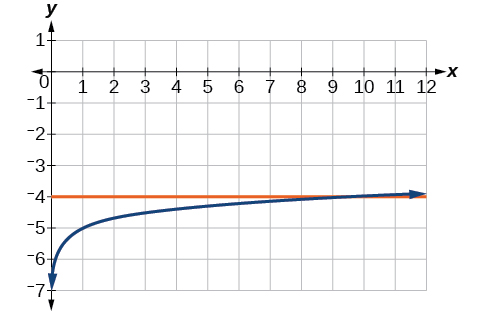${\mathrm{log}}_{3}\left(x\right)+3=2$

$\mathrm{ln}\left(3x\right)=2$

$x=\frac{{e}^{2}}{3}\approx 2.5$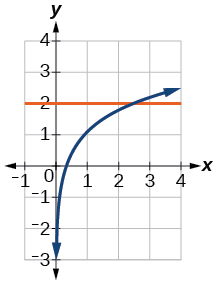$\mathrm{ln}\left(x-5\right)=1$

$\mathrm{log}\left(4\right)+\mathrm{log}\left(-5x\right)=2$

$x=-5$$-7+{\mathrm{log}}_{3}\left(4-x\right)=-6$

$\mathrm{ln}\left(4x-10\right)-6=-5$

$x=\frac{e+10}{4}\approx 3.2$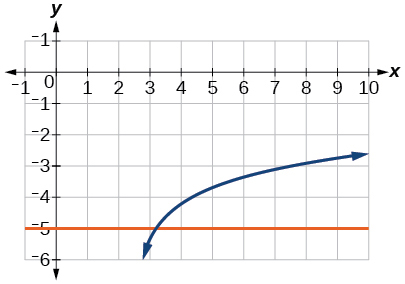$\mathrm{log}\left(4-2x\right)=\mathrm{log}\left(-4x\right)$

${\mathrm{log}}_{11}\left(-2{x}^{2}-7x\right)={\mathrm{log}}_{11}\left(x-2\right)$

No solution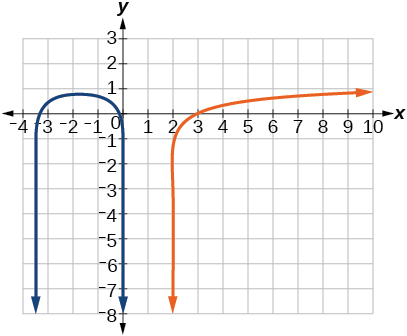$\mathrm{ln}\left(2x+9\right)=\mathrm{ln}\left(-5x\right)$

${\mathrm{log}}_{9}\left(3-x\right)={\mathrm{log}}_{9}\left(4x-8\right)$

$x=\frac{11}{5}\approx 2.2$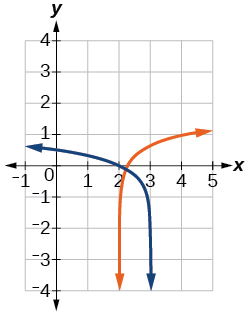$\mathrm{log}\left({x}^{2}+13\right)=\mathrm{log}\left(7x+3\right)$

$\frac{3}{{\mathrm{log}}_{2}\left(10\right)}-\mathrm{log}\left(x-9\right)=\mathrm{log}\left(44\right)$

$x=\frac{101}{11}\approx 9.2$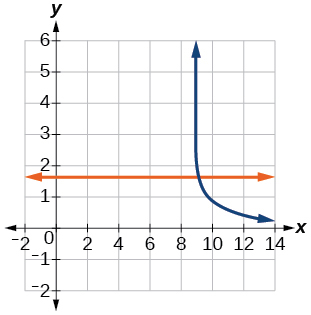$\mathrm{ln}\left(x\right)-\mathrm{ln}\left(x+3\right)=\mathrm{ln}\left(6\right)$

For the following exercises, solve for the indicated value, and graph the situation showing the solution point.

An account with an initial deposit of $\text{\hspace{0.17em}}\text{6,500}\text{\hspace{0.17em}}$ earns $\text{\hspace{0.17em}}7.25%\text{\hspace{0.17em}}$ annual interest, compounded continuously. How much will the account be worth after 20 years?

about $\text{\hspace{0.17em}}27,710.24$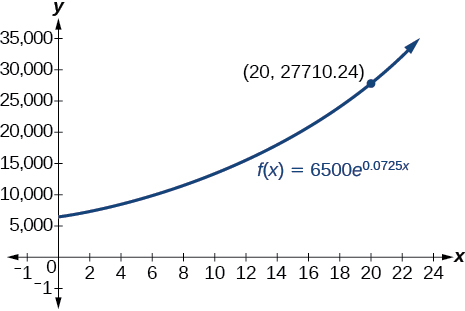The formula for measuring sound intensity in decibels $\text{\hspace{0.17em}}D\text{\hspace{0.17em}}$ is defined by the equation $\text{\hspace{0.17em}}D=10\mathrm{log}\left(\frac{I}{{I}_{0}}\right),\text{}$ where $\text{\hspace{0.17em}}I\text{\hspace{0.17em}}$ is the intensity of the sound in watts per square meter and $\text{\hspace{0.17em}}{I}_{0}={10}^{-12}\text{\hspace{0.17em}}$ is the lowest level of sound that the average person can hear. How many decibels are emitted from a jet plane with a sound intensity of $\text{\hspace{0.17em}}8.3\cdot {10}^{2}\text{\hspace{0.17em}}$ watts per square meter?

The population of a small town is modeled by the equation $\text{\hspace{0.17em}}P=1650{e}^{0.5t}\text{\hspace{0.17em}}$ where $\text{\hspace{0.17em}}t\text{\hspace{0.17em}}$ is measured in years. In approximately how many years will the town’s population reach $\text{\hspace{0.17em}}\text{20,000?}$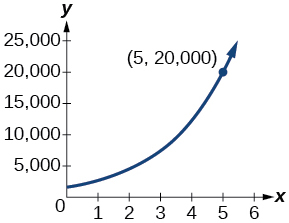## Technology

For the following exercises, solve each equation by rewriting the exponential expression using the indicated logarithm. Then use a calculator to approximate $\text{\hspace{0.17em}}x\text{\hspace{0.17em}}$ to 3 decimal places .

$1000{\left(1.03\right)}^{t}=5000\text{\hspace{0.17em}}$ using the common log.

${e}^{5x}=17\text{\hspace{0.17em}}$ using the natural log

$\frac{\mathrm{ln}\left(17\right)}{5}\approx 0.567$

$3{\left(1.04\right)}^{3t}=8\text{\hspace{0.17em}}$ using the common log

${3}^{4x-5}=38\text{\hspace{0.17em}}$ using the common log

$50{e}^{-0.12t}=10\text{\hspace{0.17em}}$ using the natural log

For the following exercises, use a calculator to solve the equation. Unless indicated otherwise, round all answers to the nearest ten-thousandth.

$7{e}^{3x-5}+7.9=47$

$x\approx 2.2401$

$\mathrm{ln}\left(3\right)+\mathrm{ln}\left(4.4x+6.8\right)=2$

$\mathrm{log}\left(-0.7x-9\right)=1+5\mathrm{log}\left(5\right)$

$x\approx -\text{44655}.\text{7143}$

Atmospheric pressure $\text{\hspace{0.17em}}P\text{\hspace{0.17em}}$ in pounds per square inch is represented by the formula $\text{\hspace{0.17em}}P=14.7{e}^{-0.21x},$ where $x$ is the number of miles above sea level. To the nearest foot, how high is the peak of a mountain with an atmospheric pressure of $\text{\hspace{0.17em}}8.369\text{\hspace{0.17em}}$ pounds per square inch? ( Hint : there are 5280 feet in a mile)

The magnitude M of an earthquake is represented by the equation $\text{\hspace{0.17em}}M=\frac{2}{3}\mathrm{log}\left(\frac{E}{{E}_{0}}\right)\text{\hspace{0.17em}}$ where $\text{\hspace{0.17em}}E\text{\hspace{0.17em}}$ is the amount of energy released by the earthquake in joules and $\text{\hspace{0.17em}}{E}_{0}={10}^{4.4}\text{\hspace{0.17em}}$ is the assigned minimal measure released by an earthquake. To the nearest hundredth, what would the magnitude be of an earthquake releasing $\text{\hspace{0.17em}}1.4\cdot {10}^{13}\text{\hspace{0.17em}}$ joules of energy?

about $\text{\hspace{0.17em}}5.83$

## Extensions

Use the definition of a logarithm along with the one-to-one property of logarithms to prove that $\text{\hspace{0.17em}}{b}^{{\mathrm{log}}_{b}x}=x.$

Recall the formula for continually compounding interest, $\text{\hspace{0.17em}}y=A{e}^{kt}.\text{\hspace{0.17em}}$ Use the definition of a logarithm along with properties of logarithms to solve the formula for time $\text{\hspace{0.17em}}t\text{\hspace{0.17em}}$ such that $\text{\hspace{0.17em}}t\text{\hspace{0.17em}}$ is equal to a single logarithm.

$t=\mathrm{ln}\left({\left(\frac{y}{A}\right)}^{\frac{1}{k}}\right)$

Recall the compound interest formula $\text{\hspace{0.17em}}A=a{\left(1+\frac{r}{k}\right)}^{kt}.\text{\hspace{0.17em}}$ Use the definition of a logarithm along with properties of logarithms to solve the formula for time $\text{\hspace{0.17em}}t.$

Newton’s Law of Cooling states that the temperature $\text{\hspace{0.17em}}T\text{\hspace{0.17em}}$ of an object at any time t can be described by the equation $\text{\hspace{0.17em}}T={T}_{s}+\left({T}_{0}-{T}_{s}\right){e}^{-kt},$ where $\text{\hspace{0.17em}}{T}_{s}\text{\hspace{0.17em}}$ is the temperature of the surrounding environment, $\text{\hspace{0.17em}}{T}_{0}\text{\hspace{0.17em}}$ is the initial temperature of the object, and $\text{\hspace{0.17em}}k\text{}$ is the cooling rate. Use the definition of a logarithm along with properties of logarithms to solve the formula for time $\text{\hspace{0.17em}}t\text{\hspace{0.17em}}$ such that $\text{\hspace{0.17em}}t\text{\hspace{0.17em}}$ is equal to a single logarithm.

$t=\mathrm{ln}\left({\left(\frac{T-{T}_{s}}{{T}_{0}-{T}_{s}}\right)}^{-\text{\hspace{0.17em}}\frac{1}{k}}\right)$

#### Questions & Answers

write down the polynomial function with root 1/3,2,-3 with solution
if A and B are subspaces of V prove that (A+B)/B=A/(A-B)
write down the value of each of the following in surd form a)cos(-65°) b)sin(-180°)c)tan(225°)d)tan(135°)
Prove that (sinA/1-cosA - 1-cosA/sinA) (cosA/1-sinA - 1-sinA/cosA) = 4
what is the answer to dividing negative index
In a triangle ABC prove that. (b+c)cosA+(c+a)cosB+(a+b)cisC=a+b+c.
give me the waec 2019 questions
the polar co-ordinate of the point (-1, -1)
prove the identites sin x ( 1+ tan x )+ cos x ( 1+ cot x )= sec x + cosec x
tanh`(x-iy) =A+iB, find A and B
B=Ai-itan(hx-hiy)
Rukmini
what is the addition of 101011 with 101010
If those numbers are binary, it's 1010101. If they are base 10, it's 202021.
Jack
extra power 4 minus 5 x cube + 7 x square minus 5 x + 1 equal to zero
the gradient function of a curve is 2x+4 and the curve passes through point (1,4) find the equation of the curve
1+cos²A/cos²A=2cosec²A-1
test for convergence the series 1+x/2+2!/9x3ByByBy